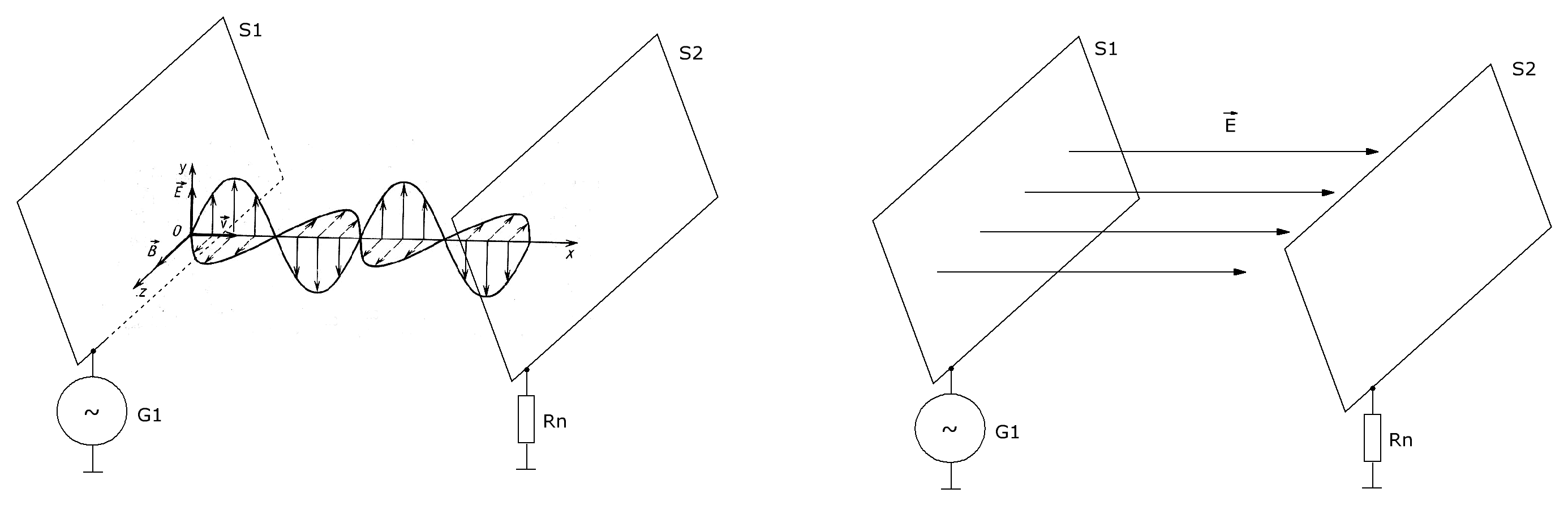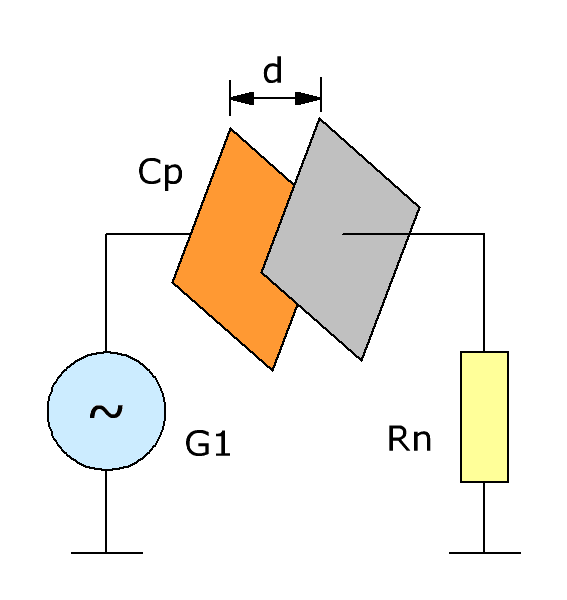Research website of Vyacheslav Gorchilin
2019-01-16
Electrodynamic induction. The radio on EDIE

The first class of effects I'm going to show You is the effects produced by electrostatic force. Is the force that governs the movement of atoms, leads to their collision, and generates an energy of heat and light. This force also causes the aggregation of atoms, an infinite number of ways in accordance with the fantastic designs of Nature, and forms all those amazing structures that we see around us.

N. Tesla. The lecture "On light and other high frequency phenomena"

There are several ways of transferring the electric potential at some distance, but in this work we will only consider two of them: electromagnetic and electrostatic (Fig. 1). In fact, always involved both, but depending on experimental conditions one of them can prevail. You can even call these methods as Gerzofsky and Coulomb, as in the first case, the potential is transferred by waves of Hertz, and the second — with the Coulomb field. The transfer of electromagnetic wave is studied well enough, for he developed quite a strong mathematical apparatus and it is used in almost all areas of radio engineering. We will allow only for comparison with the second transfer method — electric field, more precisely a field of charges, which still remains a mystery even for professionals. A little "raise the curtain" we will put a few experiments that clearly show this method in action and then compare with the first.Fig.1. The transfer of capacity from S1 to S2 electromagnetic wave (left) and electric field (right)
According to Maxwell's theory, when the electric field always should be a vortex magnetic field. In our work, we will investigate the relatively low-frequency changes, in which the magnetic field is negligible even when the theoretical calculation. In addition, there is every reason to believe that, in practice, inside the capacitor, magnetic fields do not occur [2,3]. Insights from these works to support the work of Tesla transformer, high voltage end of which is connected to a remote container, which, in turn, forms a capacitor with the surface of the Earth. Because of the quarter-wave distribution around the container, we can observe only an electric field, and magnetic — formed in current (earth) side of this transformer.
1. Electrodynamic induction and displacement current
Maxwell's equations considers two types of current: conduction current and displacement current. First of all know very well, it flows through any conductive materials, in its motion forms a magnetic field and it can be measured by an ordinary ammeter. But what if the path of the current occurs in the dielectric? Because the conduction current it cannot flow.Fig.2. Electric RC-circuit for alternating current
However, we know that in an AC circuit with a series connected generator G1, a capacitor Cp and a resistance Rn, for the past is fixed, the potential difference (Fig. 2). How? Here comes to the rescue and the bias current, which occurs when any change of the electric field in time , it then "passes" through a capacitor.
Ie, in AC circuits one species into another quite naturally, so that we don't even think about it and in the calculation of such circuits, we believe that it is one and the same current!
The term "electrodynamic induction" was introduced by D. A. Dudkina [4,5] on the basis of his experiments with moving charged bodies. Mostly it is a smooth transition from stationary to dynamic electrostatics, in fact, a new direction in physics. It is interesting what processes occur when the electric field in time, i.e., by definition, involves the displacement currents. In their experiments, we will show that from the viewpoint of migration potential, the processes are exactly the same: whether the charged moving body relative to an unloaded or static body, but one of the bodies is charged and discharged with some period. But this is what we observe in the capacitor Cp in Fig. 2. Therefore, in this work (and beyond) we will use the term "electrodynamic induction" (EDI), as an analogue of the bias current applied to the electrostatic transfer method potential.
Neither Fig. 1 introduced two ways of transferring capacity, now the second one can be called more precisely: the transfer of electrodynamic induction. If the first method is used for this transverse wave, the second, apparently, is longitudinal, which is now also many legends. But a grain of truth in this, because mathematical it only began to be supplied , and many of its parameters is simply unknown, for example, the speed of propagation of such waves, the ability of its passage through the different environments, etc.
But the dependence of the amplitude of the potential on the distance between the transmitter and the receiver we can estimate. The capacitance of the capacitor Cp (Fig. 2) decreases in proportion to the distance between the plates (d), consequently the difference of potentials on Rn must be inversely proportional to this distance. Interestingly, the same dependence is observed for transverse waves, where the voltage at the receiving antenna is inversely proportional to the distance from the transmitting (in far zone).
Next, we will show you how to make the necessary tools for fixing EDI and carry out several experiments on the "dynamic electrostatic", and later repeat the experiments Tesla, Popov and Marconi via electrostatic bonds.

1 2 3The materials used
1. Wikipedia. A bias current.
2. V. S. Gudymenko, V. I. Piskunov. Experimental verification of the existence of a magnetic field generated by the bias currents of the capacitor.
3. Zadorozhnyi V. N. Bias current and magnetic field.
4. Dudkin D. A. Scientific discovery of electrodynamic induction.
5. Dudkin D. A., Komarov A. A. Electrodynamic induction. A new concept of Geomagnetism // Preprint NASU, Donipht-01-01, 2001. – 70 p
6. A. K. Tomilin. The fundamentals of generalized electrodynamics.